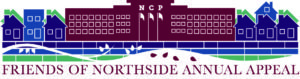# Annual Appeal Letters are in the Mail

Please check your mailbox for an important Annual Appeal letter from Principal Mest. It explains why annual giving is essential to maintaining Northside's excellent reputation and how Annual Appeal giving contributes directly to the educational experience of your child.

The mailing includes a form which outlines methods and levels of giving. Our parent volunteers have been working hard to ensure that your giving experience goes as smoothly as possible. You have many choices: check, money order, online payment with credit card, gift of stock shares or securities, monthly giving-we can handle it all!

Want to donate now? Click HERE. All donations are tax deductible, and any amount is greatly appreciated!

 table div table+table+table div table{width:100%;padding:0}table div table+table+table div table img{width:96.23%;padding:0;float:none}table div table+table+table div table td{width:100%;padding:0 1.88% 18px}/* styles */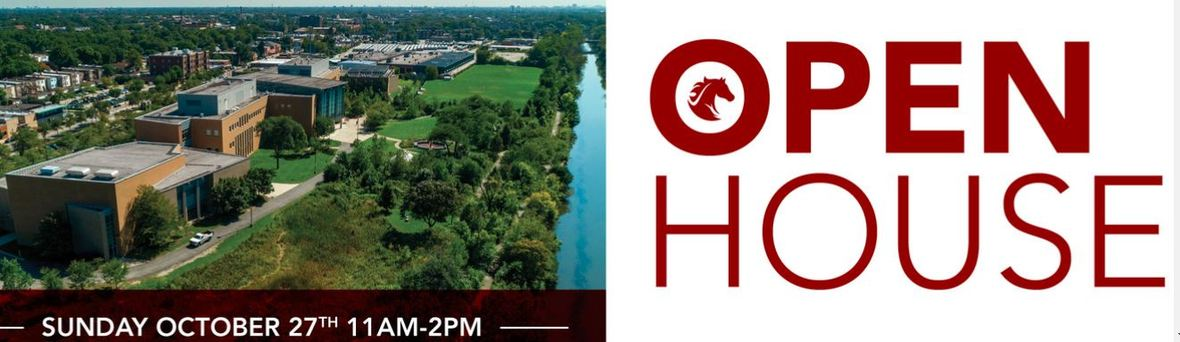# Northside's Selective Enrollment Open House

## Student and Parent Volunteers Needed

Sunday, October 27
11:00 am - 2:00 pm

Please encourage your students to sign up to help with the Open House. Student volunteers are needed from 9:00 am - 3:00 pm. There will be two training sessions: Wednesday, October 9 and Wednesday, October 23. Students will be able to pick from flex 2 and flex 4 sessions. On the day of the Open House, students will show families around, answer questions, and have the opportunity to share what they love about Northside. Food and snacks will be provided and students will earn service learning hours. Please encourage students to fill out this FORM to sign up.

We also need 100 adult volunteers to work this busy but super-fun event. Parents help answer questions and direct crowds in the gym, outside and all over the building. You will need to be registered as a Level II volunteer if you have not done so already. It very easy to do - you must complete a form and turn in an ID. Please see details below under CPS Volunteer Requirements.

 table div table+table+table+table+table+table div table{width:100%;padding:0}table div table+table+table+table+table+table div table img{width:96.23%;padding:0;float:none}table div table+table+table+table+table+table div table td{width:100%;padding:0 1.88% 18px}/* styles */# Yearbook Portrait Retakes

Wednesday, October 9
10:00 am - 3:30 pm

Portrait retakes are this Wednesday, October 9 from 10:00 am - 3:30 pm during colloquium. Stuart-Rodgers will have their photographers set up in the third-floor atrium. It is the students' responsibility to have retakes done during this time. Please ask them not to wait until the very end of colloquium - the photographers will run out of time and their portrait will not be taken.

A yearbook sitting is free, but if you wish to order portrait packages, your student will need to turn in an order form available HERE with payment when they have their portrait taken.

 table div table+table+table+table+table+table+table+table div table{width:100%;padding:0}table div table+table+table+table+table+table+table+table div table img{width:96.23%;padding:0;float:none}table div table+table+table+table+table+table+table+table div table td{width:100%;padding:0 1.88% 18px}/* styles */# Volunteers Needed for Spanish Honor Society

Wednesday, October 9 & Wednesday, October 30
8:00 am - 9:30 am

For the past 10 years, Northside's award-winning Spanish Honor Society has gone to Hibbard Elementary nearby to read to and tutor first graders who are Spanish speakers. It's a great volunteer opportunity for the students to practice their Spanish outside of class, but we need chaperones!

One chaperone is needed for Wednesday, October 9 from 8:00 am - 9:30 am and one for Wednesday, October 30 from 8:00 am - 9:30 am.

Please contact Señor Blease if you can walk with the students. It's less than a mile away.

 table div table+table+table+table+table+table+table+table+table+table div table{width:100%;padding:0}table div table+table+table+table+table+table+table+table+table+table div table img{width:96.23%;padding:0;float:none}table div table+table+table+table+table+table+table+table+table+table div table td{width:100%;padding:0 1.88% 18px}/* styles */# Next Monday - No School - CPS Day Off

Monday, October 14
Indigenous People's Day/Columbus Day

 table div table+table+table+table+table+table+table+table+table+table+table+table div table{width:100%;padding:0}table div table+table+table+table+table+table+table+table+table+table+table+table div table img{width:96.23%;padding:0;float:none}table div table+table+table+table+table+table+table+table+table+table+table+table div table td{width:100%;padding:0 1.88% 18px}/* styles */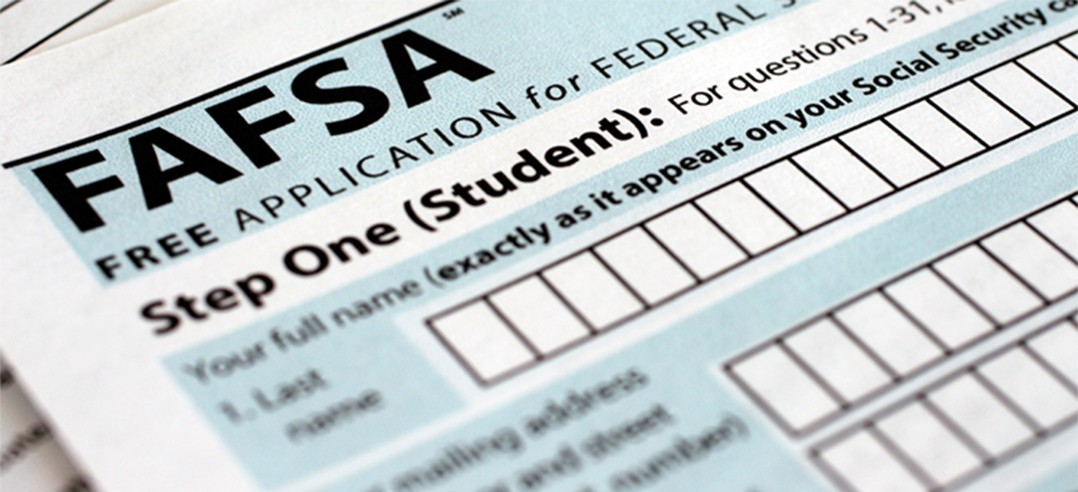# Financial Aid Completion Workshops

ISAC Parent and Student FAFSA Workshop (English & Spanish)
Tuesday, October 15
6:00 - 8:00 pm
Room 219

ISAC Student Financial Aid Follow-up
Wednesday, November 20
Two sessions: 1:25 - 2:10 pm and 2:15 - 3:00 pm
Room 224

The Free Application for Federal Student Aid (FAFSA) launch date for the 2020-2021 academic year was October 1. The Northside Prep Counseling Department will offer FAFSA Completion Workshops for English and Spanish-speaking parents and students on Tuesday, October 15 from 6:00 - 8:00 pm.

Additional student workshops will be held during the day for students who would like to start their FAFSA or if they would like one-on-one support.

Students and parents, please register HERE for the FAFSA Workshops. Students will be required to register for the workshops offered during the school day in their Enriching Student Portal as well.

Also, ISAC will host two financial aid follow-up presentations on Wednesday, November 20 to address what happens after a FAFSA is completed. Some of the topics will include reviewing the Student Aid Report (SAR), completing the federal income verification process, and using the IRS Data Retrieval Tool. Sessions will be held at 1:25 - 2:10 pm and 2:15 - 3:00 pm.

In addition to the FAFSA Completion Workshops, the counseling department has shared some useful resources below that may assist you and your family with navigating the next steps in the financial aid process.

▪ The FAFSA Process Graphic by the Federal Student Aid.
▪ 7 Things You Need Before You Fill Out the 2020–21 FAFSA® Form, (2019) by the Federal Student Aid.
▪ FAFSA Tips & Common Mistakes to Avoid (2018) by the National Association of Student Financial Aid Administrators.
▪ 8 Things to Do After Filing Your FAFSA Form (2019) by Chicago Public Schools Office of School Counseling and Postsecondary Advising and the Office of College and Career Success.
 ▪ The FAFSA Process Graphic by the Federal Student Aid.
 ▪ 7 Things You Need Before You Fill Out the 2020–21 FAFSA® Form, (2019) by the Federal Student Aid.
 ▪ FAFSA Tips & Common Mistakes to Avoid (2018) by the National Association of Student Financial Aid Administrators.
 ▪ 8 Things to Do After Filing Your FAFSA Form (2019) by Chicago Public Schools Office of School Counseling and Postsecondary Advising and the Office of College and Career Success.
 table div table+table+table+table+table+table+table+table+table+table+table+table+table+table div table{width:100%;padding:0}table div table+table+table+table+table+table+table+table+table+table+table+table+table+table div table img{width:96.23%;padding:0;float:none}table div table+table+table+table+table+table+table+table+table+table+table+table+table+table div table td{width:100%;padding:0 1.88% 18px}/* styles */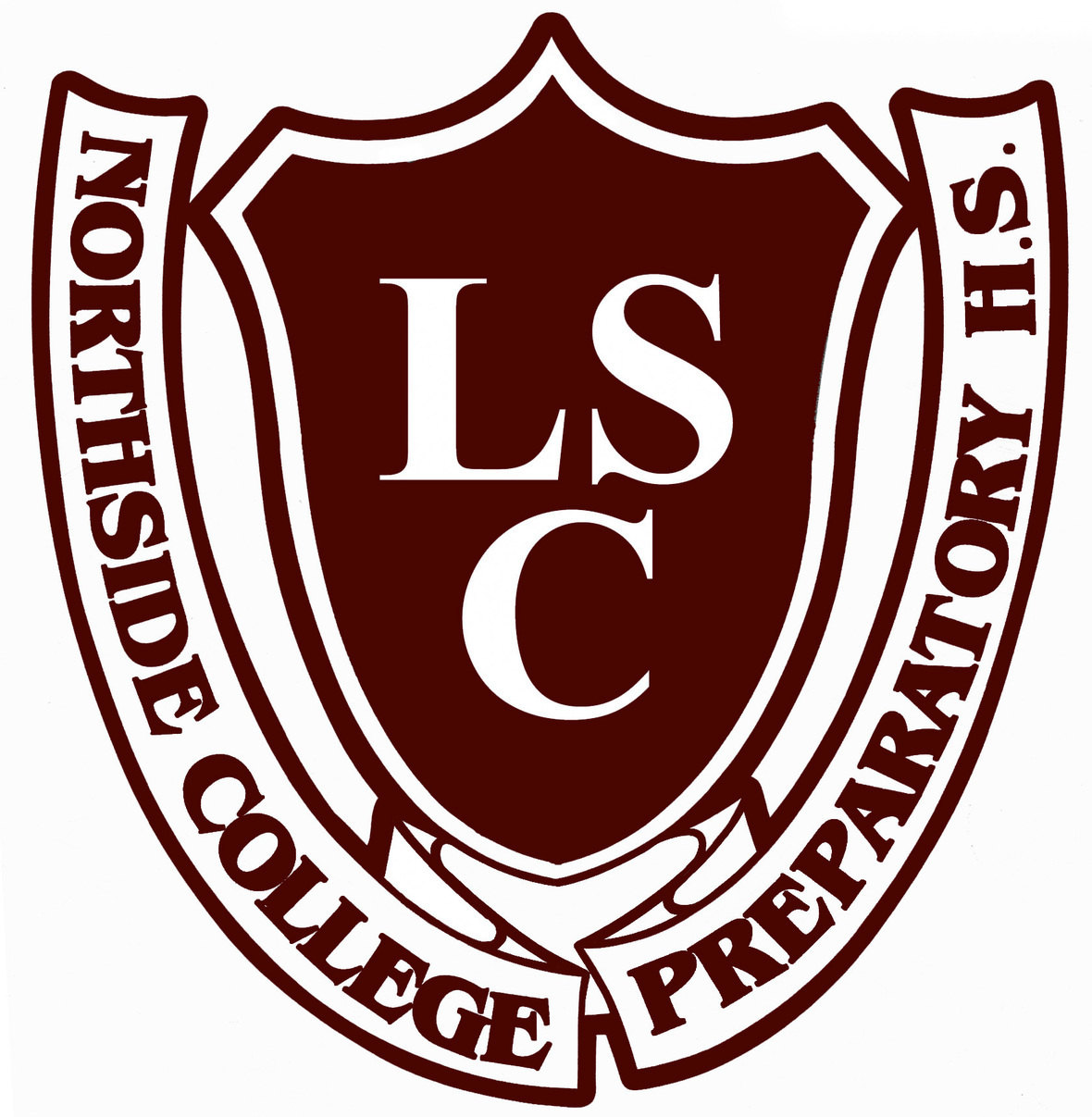# Local School Council (LSC) Meeting

Tuesday, October 15
6:30 pm
Faculty Lounge

 table div table+table+table+table+table+table+table+table+table+table+table+table+table+table+table+table div table{width:100%;padding:0}table div table+table+table+table+table+table+table+table+table+table+table+table+table+table+table+table div table img{width:96.23%;padding:0;float:none}table div table+table+table+table+table+table+table+table+table+table+table+table+table+table+table+table div table td{width:100%;padding:0 1.88% 18px}/* styles */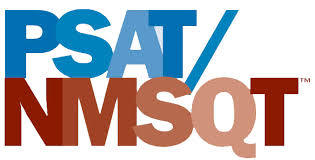# PSAT/NMST Exam

Wednesday, October 16
8:00 am

Northside College Prep sophomores and juniors will participate in the PSAT/NMSQT exam. On the day of the exam, students are expected to report to school no later than 8:00 am for a full day of school. More details about the exam will be shared with students and parents in the near future.

 table div table+table+table+table+table+table+table+table+table+table+table+table+table+table+table+table+table+table div table{width:100%;padding:0}table div table+table+table+table+table+table+table+table+table+table+table+table+table+table+table+table+table+table div table img{width:96.23%;padding:0;float:none}table div table+table+table+table+table+table+table+table+table+table+table+table+table+table+table+table+table+table div table td{width:100%;padding:0 1.88% 18px}/* styles */# FON Parent College Night

Thursday, October 17
6:30 - 8:00 pm
Second Floor Atrium

The Friends of Northside sponsored Parent College Night will be held Thursday, October 17 from 6:30 - 8:00 pm in the Second Floor Atrium.

Come hear parents of recently graduated NCP students share their experiences and advice about the college search and application process. Please RSVP for the event HERE so we know how many people to expect.

 table div table+table+table+table+table+table+table+table+table+table+table+table+table+table+table+table+table+table+table+table div table{width:100%;padding:0}table div table+table+table+table+table+table+table+table+table+table+table+table+table+table+table+table+table+table+table+table div table img{width:96.23%;padding:0;float:none}table div table+table+table+table+table+table+table+table+table+table+table+table+table+table+table+table+table+table+table+table div table td{width:100%;padding:0 1.88% 18px}/* styles */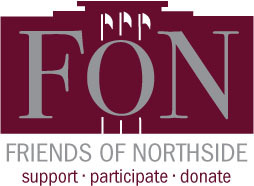# Friends of Northside Meeting

Tuesday, October 22
7:00 pm
Faculty Lounge

 table div table+table+table+table+table+table+table+table+table+table+table+table+table+table+table+table+table+table+table+table+table+table div table{width:100%;padding:0}table div table+table+table+table+table+table+table+table+table+table+table+table+table+table+table+table+table+table+table+table+table+table div table img{width:96.23%;padding:0;float:none}table div table+table+table+table+table+table+table+table+table+table+table+table+table+table+table+table+table+table+table+table+table+table div table td{width:100%;padding:0 1.88% 18px}/* styles */# AP Exam Information: Deadline to Register is Friday, November 15

Students taking AP exams at Northside College Prep HS must register HERE and pay by 4:00 pm on Friday, November 15, 2019.

For the 2020 AP Exams, the cost is \$110 per exam and \$15 if your student qualifies for a free/reduced lunch. Please adhere to the November 15 deadline as there will be a mandatory College Board late fee of \$40.00 charged in addition to the exam fee. Please contact Mr. Alexander Hughes if you have any additional questions.

 table div table+table+table+table+table+table+table+table+table+table+table+table+table+table+table+table+table+table+table+table+table+table+table+table+table div table{width:100%;padding:0}table div table+table+table+table+table+table+table+table+table+table+table+table+table+table+table+table+table+table+table+table+table+table+table+table+table div table img{width:96.23%;padding:0;float:none}table div table+table+table+table+table+table+table+table+table+table+table+table+table+table+table+table+table+table+table+table+table+table+table+table+table div table td{width:100%;padding:0 1.88% 18px}/* styles */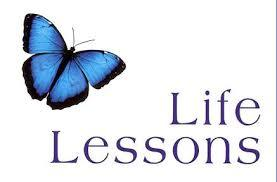# Guest Speakers Needed for Life Lessons Colloquium

Mr. Madia is looking for parents who would like to be a guest speaker for his life lessons colloquium.

He's looking for people in the field of finance, cooking, fashion, travel, hiring manager, auto, housing, life skills, etc. Please contact Mr. Madia directly to help.

 table div table+table+table+table+table+table+table+table+table+table+table+table+table+table+table+table+table+table+table+table+table+table+table+table+table+table+table div table{width:100%;padding:0}table div table+table+table+table+table+table+table+table+table+table+table+table+table+table+table+table+table+table+table+table+table+table+table+table+table+table+table div table img{width:96.23%;padding:0;float:none}table div table+table+table+table+table+table+table+table+table+table+table+table+table+table+table+table+table+table+table+table+table+table+table+table+table+table+table div table td{width:100%;padding:0 1.88% 18px}/* styles */# Donations Needed for Student Resource Closet

Friends of Northside has a resource closet available to NCP students. Currently, we are in need of:

▪ Individual snacks such as granola bars, nuts, and dried fruit
▪ Toothpaste and dental floss
▪ Hair conditioner
▪ Men’s and women’s underwear and socks
▪ Loose leaf and graph paper
▪ Spiral notebooks and binders
▪ Colored pencils
▪ Sanitizing wipes (Clorox type)
▪ Dryer sheets
▪ Laundry soap
 ▪ Individual snacks such as granola bars, nuts, and dried fruit
 ▪ Toothpaste and dental floss
 ▪ Hair conditioner
 ▪ Men’s and women’s underwear and socks
 ▪ Loose leaf and graph paper
 ▪ Spiral notebooks and binders
 ▪ Colored pencils
 ▪ Sanitizing wipes (Clorox type)
 ▪ Dryer sheets
 ▪ Laundry soap

All items must be new and unopened. No used clothing will be accepted. Please bring all items to the Counseling Office and set outside of Ms. Matthews' office.

Contact Nancy Liljedahl with any questions.

 table div table+table+table+table+table+table+table+table+table+table+table+table+table+table+table+table+table+table+table+table+table+table+table+table+table+table+table+table+table+table div table{width:100%;padding:0}table div table+table+table+table+table+table+table+table+table+table+table+table+table+table+table+table+table+table+table+table+table+table+table+table+table+table+table+table+table+table div table img{width:96.23%;padding:0;float:none}table div table+table+table+table+table+table+table+table+table+table+table+table+table+table+table+table+table+table+table+table+table+table+table+table+table+table+table+table+table+table div table td{width:100%;padding:0 1.88% 18px}/* styles */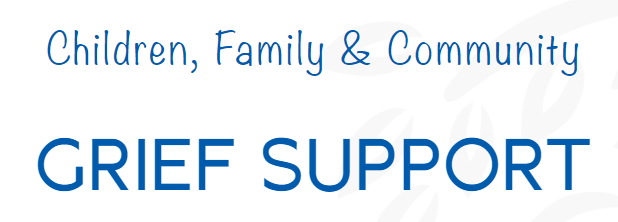/* styles */ If parents are interested in learning more about how to support their children through grief, they can reach out to Willow House for support. Last school year, Willow House provided grief support at Northside for students and staff and also offered staff training. They can provide expert guidance for parents on what to expect for adolescent grief, how to support your child, and when to seek out additional help. All services are free of charge. Contact Willow House or Anne Cooper, NCP social worker, with any questions.
 table div table+table+table+table+table+table+table+table+table+table+table+table+table+table+table+table+table+table+table+table+table+table+table+table+table+table+table+table+table+table+table+table+table div table{width:100%;padding:0}table div table+table+table+table+table+table+table+table+table+table+table+table+table+table+table+table+table+table+table+table+table+table+table+table+table+table+table+table+table+table+table+table+table div table img{width:96.23%;padding:0;float:none}table div table+table+table+table+table+table+table+table+table+table+table+table+table+table+table+table+table+table+table+table+table+table+table+table+table+table+table+table+table+table+table+table+table div table td{width:100%;padding:0 1.88% 18px}/* styles */# Important October Dates

▪ Wednesday, October 9 - Yearbook portrait retakes
▪ Monday, October 14 - Indigenous People's Day/Columbus Day (no school)
▪ Tuesday, October 15 - LSC Meeting & ISAC Parent and Student FAFSA Workshop (English & Spanish)
▪ Wednesday, October 16 - PSAT/NMST Exam
▪ Thursday, October 17 - FON Parent College Night & Athletic Booster Club meeting
▪ Friday, October 18 - Latino Fest
▪ Tuesday, October 22 - FON Meeting
▪ Friday, October 25 - Halloween Dance (sponsored by the Latin Club)
▪ Sunday, October 27 - NCP Open House
▪ Tuesday, October 29 - Halloween Concert
 ▪ Wednesday, October 9 - Yearbook portrait retakes
 ▪ Monday, October 14 - Indigenous People's Day/Columbus Day (no school)
 ▪ Tuesday, October 15 - LSC Meeting & ISAC Parent and Student FAFSA Workshop (English & Spanish)
 ▪ Wednesday, October 16 - PSAT/NMST Exam
 ▪ Thursday, October 17 - FON Parent College Night & Athletic Booster Club meeting
 ▪ Friday, October 18 - Latino Fest
 ▪ Tuesday, October 22 - FON Meeting
 ▪ Friday, October 25 - Halloween Dance (sponsored by the Latin Club)
 ▪ Sunday, October 27 - NCP Open House
 ▪ Tuesday, October 29 - Halloween Concert
 table div table+table+table+table+table+table+table+table+table+table+table+table+table+table+table+table+table+table+table+table+table+table+table+table+table+table+table+table+table+table+table+table+table+table+table+table div table{width:100%;padding:0}table div table+table+table+table+table+table+table+table+table+table+table+table+table+table+table+table+table+table+table+table+table+table+table+table+table+table+table+table+table+table+table+table+table+table+table+table div table img{width:96.23%;padding:0;float:none}table div table+table+table+table+table+table+table+table+table+table+table+table+table+table+table+table+table+table+table+table+table+table+table+table+table+table+table+table+table+table+table+table+table+table+table+table div table td{width:100%;padding:0 1.88% 18px}/* styles */# This Week in Sports

Come out and support our student athletes!

## Women's Swimming

▪ Thursday, October 10, 5:00pm (V) vs. Von Steuben at NCP
 ▪ Thursday, October 10, 5:00pm (V) vs. Von Steuben at NCP
 /* styles */ Visit the NCP Athletics website for additional information about Northside teams.
 table div table+table+table+table+table+table+table+table+table+table+table+table+table+table+table+table+table+table+table+table+table+table+table+table+table+table+table+table+table+table+table+table+table+table+table+table+table+table+table div table{width:100%;padding:0}table div table+table+table+table+table+table+table+table+table+table+table+table+table+table+table+table+table+table+table+table+table+table+table+table+table+table+table+table+table+table+table+table+table+table+table+table+table+table+table div table img{width:96.23%;padding:0;float:none}table div table+table+table+table+table+table+table+table+table+table+table+table+table+table+table+table+table+table+table+table+table+table+table+table+table+table+table+table+table+table+table+table+table+table+table+table+table+table+table div table td{width:100%;padding:0 1.88% 18px}/* styles */# No Experience Necessary

Make a commitment to Northside by offering your time for any of these rewarding on-going volunteer positions. Email Friends of Northside to indicate your interest or ask questions.

## Graphic Designer

Provide professional guidance and materials for various design needs throughout the year.

## Volunteer Coordinator

Manage and update our online volunteer system as needed throughout the year.

## Spring Benefit Committee

The Spring Benefit is NCP’s major spring fundraiser. Get involved, get to know NCP staff and parents, and make a profound impact on Northside College Prep.

When is the Spring Benefit?
Friday, March 13, 2020

What’s the time commitment for the team?

Email Debra Swan, Event Chair, for more information or to join the team.

 table div table+table+table+table+table+table+table+table+table+table+table+table+table+table+table+table+table+table+table+table+table+table+table+table+table+table+table+table+table+table+table+table+table+table+table+table+table+table+table+table+table+table+table div table{width:100%;padding:0}table div table+table+table+table+table+table+table+table+table+table+table+table+table+table+table+table+table+table+table+table+table+table+table+table+table+table+table+table+table+table+table+table+table+table+table+table+table+table+table+table+table+table+table div table img{width:96.23%;padding:0;float:none}table div table+table+table+table+table+table+table+table+table+table+table+table+table+table+table+table+table+table+table+table+table+table+table+table+table+table+table+table+table+table+table+table+table+table+table+table+table+table+table+table+table+table+table div table td{width:100%;padding:0 1.88% 18px}/* styles */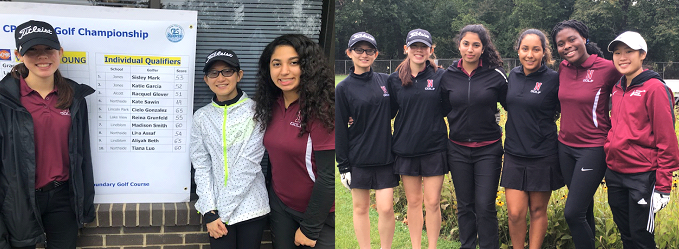# NCP Girls Golf Pulled Off a Great Season!

Huge congratulations go out to the amazing six senior girls who made up this year's NCP Women's Varsity Golf Team. While the three veterans of Tiana Luo, Lina Assaf, and Kate Sawin were poised to finish out their final year of NCP golf, they found themselves short of a team when the season began. With the eagerness and thoughtfulness of three brand new senior golfers in Destiny Lara, Nasya Danso, and Rachel Vu, the Women's Varsity Golf Team could continue their season and go on to compete in the CPS City Championship, where Kate Sawin finished first in the CPS Individual competition. The new players saved the season and everyone bonded as a wonderful group of girls. Way to go, Mustangs!

If your daughter is interested in NCP Girl's Golf for next September, please reach out to Kate Sawin and the senior girls will mentor the new players prior to next year's season.

# Do you have news or team results to share with the Northside parent community?

 table div table+table+table+table+table+table+table+table+table+table+table+table+table+table+table+table+table+table+table+table+table+table+table+table+table+table+table+table+table+table+table+table+table+table+table+table+table+table+table+table+table+table+table+table+table+table+table+table div table{width:100%;padding:0}table div table+table+table+table+table+table+table+table+table+table+table+table+table+table+table+table+table+table+table+table+table+table+table+table+table+table+table+table+table+table+table+table+table+table+table+table+table+table+table+table+table+table+table+table+table+table+table+table div table img{width:96.23%;padding:0;float:none}table div table+table+table+table+table+table+table+table+table+table+table+table+table+table+table+table+table+table+table+table+table+table+table+table+table+table+table+table+table+table+table+table+table+table+table+table+table+table+table+table+table+table+table+table+table+table+table+table div table td{width:100%;padding:0 1.88% 18px}/* styles */# October College Visits at Northside

Monday, October 7
University of California, Davis, 9:48 am
Franklin University Switzerland, 9:48 am
Swarthmore College, 9:48 am
Brock University, 10:38 am
University of Illinois at Chicago, 10:38 am
Monmouth College, 12:26 pm
Occidental College,12:26 pm
Soka University of America, 3:15 pm

Tuesday, October 8
University of California, San Diego, 9:48 am
Florida Institute of Technology, 9:48 am
Michigan State University, 9:48 am
University of Wisconsin, Madison, 3:15 pm

Wednesday, October 9
Stanford University, 10:40 am
Kansas City Art Institute, 11:20 am
University of California, Los Angeles, 12:40 pm
Pennsylvania State University, 1:25 pm

Thursday, October 10
Cedarville University, 9:48 am
Calvin University, 11:36 am
University of Utah, 11:36 am
Purdue University, 12:26 pm

Friday, October 11
Fashion Institute of Design and Merchandising, Los Angeles, 10:15 am
Ferris State University, 10:15 am
Michigan Technological University, 10:15 am
University of South Carolina – Columbia, 10:15 am
University of Vermont, 10:15 am
University of Maryland, College Park, 10:38 am
University of California, Riverside, 3:15 pm

Tuesday, October 15
Eckerd College, 11:36 am
California College of the Arts (San Francisco),12:26 pm
Macalester College, 3:15 pm

Thursday, October 17
Champlain College, 9:48 am
Lawrence University, 9:48 am
Marquette University, 9:48 am
Northwestern University, 9:48 am
Earlham College, 10:38 am
Rutgers University-New Brunswick, 10:38 am
Johns Hopkins University, 11:36 am

Friday, October 18
The University of Akron, 9:48 am
Illinois Institute of Technology, 9:48 am
Roosevelt University, 9:48 am
Vanderbilt University, 9:48 am
Haverford College,12:26 pm
Kenyon College, 3:15 pm

The list of college visits is updated frequently. For the most current information, parents of juniors and seniors should log into their parent account for Naviance.

Students are highly encouraged to meet with the reps who take the time to visit Northside. Oftentimes these are the same regional reps who will be reading your child's application! Students may ask a teacher to be briefly excused from class to meet a college representative.

 table div table+table+table+table+table+table+table+table+table+table+table+table+table+table+table+table+table+table+table+table+table+table+table+table+table+table+table+table+table+table+table+table+table+table+table+table+table+table+table+table+table+table+table+table+table+table+table+table+table+table+table div table{width:100%;padding:0}table div table+table+table+table+table+table+table+table+table+table+table+table+table+table+table+table+table+table+table+table+table+table+table+table+table+table+table+table+table+table+table+table+table+table+table+table+table+table+table+table+table+table+table+table+table+table+table+table+table+table+table div table img{width:96.23%;padding:0;float:none}table div table+table+table+table+table+table+table+table+table+table+table+table+table+table+table+table+table+table+table+table+table+table+table+table+table+table+table+table+table+table+table+table+table+table+table+table+table+table+table+table+table+table+table+table+table+table+table+table+table+table+table div table td{width:100%;padding:0 1.88% 18px}/* styles */# CPS Scholarship Notifications and College Events Around the City

For more details about the upcoming events and to access the most recent updates, view the College Calendar on the Northside Prep Counseling website HERE.

 table div table+table+table+table+table+table+table+table+table+table+table+table+table+table+table+table+table+table+table+table+table+table+table+table+table+table+table+table+table+table+table+table+table+table+table+table+table+table+table+table+table+table+table+table+table+table+table+table+table+table+table+table+table div table{width:100%;padding:0}table div table+table+table+table+table+table+table+table+table+table+table+table+table+table+table+table+table+table+table+table+table+table+table+table+table+table+table+table+table+table+table+table+table+table+table+table+table+table+table+table+table+table+table+table+table+table+table+table+table+table+table+table+table div table img{width:96.23%;padding:0;float:none}table div table+table+table+table+table+table+table+table+table+table+table+table+table+table+table+table+table+table+table+table+table+table+table+table+table+table+table+table+table+table+table+table+table+table+table+table+table+table+table+table+table+table+table+table+table+table+table+table+table+table+table+table+table div table td{width:100%;padding:0 1.88% 18px}/* styles */# CHOICES College Fair: Post High School Educational Options for Students with IEPs & 504s

Tuesday, October 22
6:00-8:45 pm
New Trier High School Northfield campus

The CHOICES Program is a postsecondary planning evening for college-bound students with an IEP, 504 plan, official accommodations plan, or history of a disability.

Approximately 60 colleges, universities, and college transition programs have signed up for the CHOICES college fair on Tuesday, October 22 to meet with students and discuss the support services available at their institutions. Two breakout sessions will be offered, one at 6:00 pm and another at 6:50 pm. Topics for each session are listed below. The college fair will take place from 7:00-8:45 pm.

## 6:00-6:40 pm Breakout Session Topics

▪ Successfully and Sanely Navigating College Admission Tests - Compass Education Group
▪ Why Consider a Community College - Oakton Community College
 ▪ Successfully and Sanely Navigating College Admission Tests - Compass Education Group
 ▪ Why Consider a Community College - Oakton Community College

## 6:50-7:30 pm Breakout Session Topics

▪ Successfully and Sanely Navigating College Admission Tests - Compass Education Group
▪ The Nuts and Bolts of the Application Process - Counselors from Maine South and Stevenson High School
 ▪ Successfully and Sanely Navigating College Admission Tests - Compass Education Group
 ▪ The Nuts and Bolts of the Application Process - Counselors from Maine South and Stevenson High School

Wednesday, October 9
5:00 pm ~ NUSA's Scholarship & Financial Resource Fair

Thursday, October 10
App Closes: City Treasurer's Hispanic Heritage Month Scholarship
7:00 pm ~ Boston University Reception in Winnetka

Tuesday, October 15
App Closes: Hispanic Heritage Foundation’s Youth Awards

Thursday, October 17
6:30 pm ~ Christian College Fair

Friday, October 18
App Closes: Omega Psi Phi Fraternity, Inc. - International High School Essay Contest
App Closes: Patricia Loyd Handy Educational Scholarship

Sunday, October 20
National Portfolio Day

Monday, October 21
5:30 pm ~ Southern Methodist University: Information Session

Friday, October 25
App Closes: Horatio Alger Association - State & National Scholarships

 table div table+table+table+table+table+table+table+table+table+table+table+table+table+table+table+table+table+table+table+table+table+table+table+table+table+table+table+table+table+table+table+table+table+table+table+table+table+table+table+table+table+table+table+table+table+table+table+table+table+table+table+table+table+table+table+table div table{width:100%;padding:0}table div table+table+table+table+table+table+table+table+table+table+table+table+table+table+table+table+table+table+table+table+table+table+table+table+table+table+table+table+table+table+table+table+table+table+table+table+table+table+table+table+table+table+table+table+table+table+table+table+table+table+table+table+table+table+table+table div table img{width:96.23%;padding:0;float:none}table div table+table+table+table+table+table+table+table+table+table+table+table+table+table+table+table+table+table+table+table+table+table+table+table+table+table+table+table+table+table+table+table+table+table+table+table+table+table+table+table+table+table+table+table+table+table+table+table+table+table+table+table+table+table+table+table div table td{width:100%;padding:0 1.88% 18px}/* styles */# New to Parent Portal?

1. Obtain your student's PIN, which should be on your acceptance letter or school welcome letter.
2. Create an account HERE.
 1 Obtain your student's PIN, which should be on your acceptance letter or school welcome letter.
 2 Create an account HERE.

PINs can only be provided to the parent/guardian in person or by mail to the address on file. They cannot be provided by phone or email, or to a student. To request your PIN, please contact Sonia Fernandez in the Counseling Office.

 table div table+table+table+table+table+table+table+table+table+table+table+table+table+table+table+table+table+table+table+table+table+table+table+table+table+table+table+table+table+table+table+table+table+table+table+table+table+table+table+table+table+table+table+table+table+table+table+table+table+table+table+table+table+table+table+table+table+table div table{width:100%;padding:0}table div table+table+table+table+table+table+table+table+table+table+table+table+table+table+table+table+table+table+table+table+table+table+table+table+table+table+table+table+table+table+table+table+table+table+table+table+table+table+table+table+table+table+table+table+table+table+table+table+table+table+table+table+table+table+table+table+table+table div table img{width:96.23%;padding:0;float:none}table div table+table+table+table+table+table+table+table+table+table+table+table+table+table+table+table+table+table+table+table+table+table+table+table+table+table+table+table+table+table+table+table+table+table+table+table+table+table+table+table+table+table+table+table+table+table+table+table+table+table+table+table+table+table+table+table+table+table div table td{width:100%;padding:0 1.88% 18px}/* styles */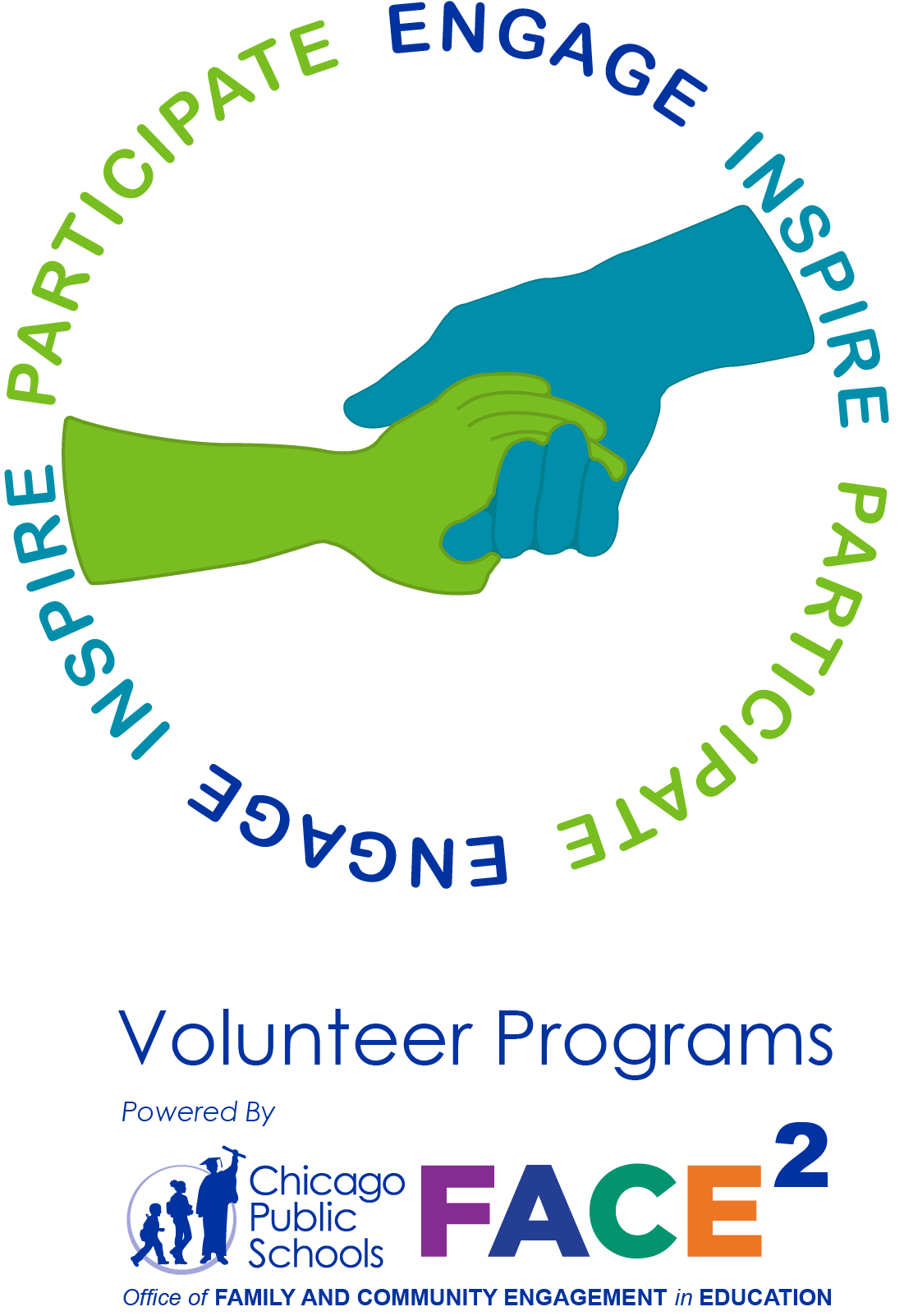# Volunteering with CPS Update: Attention Parents & Guardians Who Had Their IDs Scanned on Back-to-School Night

Ms. O'Hare has 30 scanned IDs from Back-to-School Night that do not have corresponding volunteer applications in the system. In order to approve them, she needs those parents who forgot and/or missed the step of also filling out the online application to submit their application HERE.

 table div table+table+table+table+table+table+table+table+table+table+table+table+table+table+table+table+table+table+table+table+table+table+table+table+table+table+table+table+table+table+table+table+table+table+table+table+table+table+table+table+table+table+table+table+table+table+table+table+table+table+table+table+table+table+table+table+table+table+table+table div table{width:100%;padding:0}table div table+table+table+table+table+table+table+table+table+table+table+table+table+table+table+table+table+table+table+table+table+table+table+table+table+table+table+table+table+table+table+table+table+table+table+table+table+table+table+table+table+table+table+table+table+table+table+table+table+table+table+table+table+table+table+table+table+table+table+table div table img{width:96.23%;padding:0;float:none}table div table+table+table+table+table+table+table+table+table+table+table+table+table+table+table+table+table+table+table+table+table+table+table+table+table+table+table+table+table+table+table+table+table+table+table+table+table+table+table+table+table+table+table+table+table+table+table+table+table+table+table+table+table+table+table+table+table+table+table+table div table td{width:100%;padding:0 1.88% 18px}/* styles */# CPS Volunteer Requirements

According to CPS policy, anybody who is going to be in contact with students must be approved as a CPS volunteer. There are two levels of volunteers, Level 1 and Level 2.

## Level I Volunteers

(Please allow 8-12 weeks for the entire process)
A fingerprint criminal background check is required for all Level I Volunteers.

A Level I volunteer is:

1. A parent providing volunteer service in their child’s school or classroom for 10 or more hours/week;
2. An individual providing volunteer service for 5 or more hours/week at a school where s/he does not have a child enrolled;
3. Any individual chaperoning an overnight school-sponsored trip, regardless of the hours/week that the volunteer serves (See also the Student Travel Policy).
 1 A parent providing volunteer service in their child’s school or classroom for 10 or more hours/week;
 2 An individual providing volunteer service for 5 or more hours/week at a school where s/he does not have a child enrolled;
 3 Any individual chaperoning an overnight school-sponsored trip, regardless of the hours/week that the volunteer serves (See also the Student Travel Policy).

## Level II Volunteers

(This process can be completed in a day & is required even of volunteers for Friends of Northside events)
A fingerprint criminal background check is not required for Level II Volunteers.

A Level II Volunteer is:

1. A parent providing supervised volunteer service in their child’s school or classroom for less than 10 hours/week;
2. Any individual providing volunteer service for less than 5 hours/week at a school where s/he does not have a child enrolled;
3. An individual providing incidental volunteer service with no ongoing individualized interaction with a student(s) including those who speak at a class/assembly, judge academic competitions, give musical performance, participate in the “Principal for a Day” program, job shadowing event or other one-time event provided where there is direct supervision of the activity/event by regular school employees;
4. A parent accompanying his/her child’s class on a one-day field trip or another type of extracurricular activity that does not involve an overnight stay;
5. An individual providing volunteer service on projects/activities involving no or nominal contact with children (home-based volunteer activities, volunteers serving at Central or Network office).
 1 A parent providing supervised volunteer service in their child’s school or classroom for less than 10 hours/week;
 2 Any individual providing volunteer service for less than 5 hours/week at a school where s/he does not have a child enrolled;
 3 An individual providing incidental volunteer service with no ongoing individualized interaction with a student(s) including those who speak at a class/assembly, judge academic competitions, give musical performance, participate in the “Principal for a Day” program, job shadowing event or other one-time event provided where there is direct supervision of the activity/event by regular school employees;
 4 A parent accompanying his/her child’s class on a one-day field trip or another type of extracurricular activity that does not involve an overnight stay;
 5 An individual providing volunteer service on projects/activities involving no or nominal contact with children (home-based volunteer activities, volunteers serving at Central or Network office).

To become a volunteer, please visit CPS' Volunteer website and follow the instructions. There is also a link on Northside's website on the Parents page Northside Volunteers.

Assistant Principal, Kaitlin O'Hare is Northside's Volunteer Coordinator. After applying to become a volunteer, you must visit the school and show Ms. O'Hare your ID, so she can approve you to move forward in the volunteer process. For Level II volunteers, that is the only step you must take. For Level 1, you will receive further instructions from CPS.

 table div table+table+table+table+table+table+table+table+table+table+table+table+table+table+table+table+table+table+table+table+table+table+table+table+table+table+table+table+table+table+table+table+table+table+table+table+table+table+table+table+table+table+table+table+table+table+table+table+table+table+table+table+table+table+table+table+table+table+table+table+table+table div table{width:100%;padding:0}table div table+table+table+table+table+table+table+table+table+table+table+table+table+table+table+table+table+table+table+table+table+table+table+table+table+table+table+table+table+table+table+table+table+table+table+table+table+table+table+table+table+table+table+table+table+table+table+table+table+table+table+table+table+table+table+table+table+table+table+table+table+table div table img{width:96.23%;padding:0;float:none}table div table+table+table+table+table+table+table+table+table+table+table+table+table+table+table+table+table+table+table+table+table+table+table+table+table+table+table+table+table+table+table+table+table+table+table+table+table+table+table+table+table+table+table+table+table+table+table+table+table+table+table+table+table+table+table+table+table+table+table+table+table+table div table td{width:100%;padding:0 1.88% 18px}/* styles */# FON Connect

Friends of Northside uses a customer/constituent relationship management (CRM) system known as FON Connect that allows our parent volunteer organization to work from one database for events. It is not a CPS database and is completely different and separate from the CPS Parent Portal and Northside's EPay website.

FON Connect also serves as the NCP Family Directory and allows users to review their donation history, update their contact information, register for upcoming events, and more. Each parent has a separate account. Transactions, including donations, are recorded in the account of the parent who initiated the transaction or donation.

If you’re new to FON Connect, you'll need to set up a login and password. To do so:

 table div table+table+table+table+table+table+table+table+table+table+table+table+table+table+table+table+table+table+table+table+table+table+table+table+table+table+table+table+table+table+table+table+table+table+table+table+table+table+table+table+table+table+table+table+table+table+table+table+table+table+table+table+table+table+table+table+table+table+table+table+table+table+table+table div table{width:100%;padding:0}table div table+table+table+table+table+table+table+table+table+table+table+table+table+table+table+table+table+table+table+table+table+table+table+table+table+table+table+table+table+table+table+table+table+table+table+table+table+table+table+table+table+table+table+table+table+table+table+table+table+table+table+table+table+table+table+table+table+table+table+table+table+table+table+table div table img{width:96.23%;padding:0;float:none}table div table+table+table+table+table+table+table+table+table+table+table+table+table+table+table+table+table+table+table+table+table+table+table+table+table+table+table+table+table+table+table+table+table+table+table+table+table+table+table+table+table+table+table+table+table+table+table+table+table+table+table+table+table+table+table+table+table+table+table+table+table+table+table+table div table td{width:100%;padding:0 1.88% 18px}/* styles */# Parking Lot Safety

Our NCP school parking lot is a bustling place before and after school. Families dropping off and picking up students are asked to be mindful of both safety and courtesy. Please assist us by ensuring that traffic lanes stay clear during drop-off and pick-up times and by following the parking lot procedures.

# Parking Lot Procedures

ALL vehicles MUST exit onto Kedzie. You may enter the parking lot from Kedzie or Bryn Mawr, but regardless of where you enter, all cars must exit onto Kedzie. For the safety of our students and the rest of the Northside community, please do not exit onto Bryn Mawr.

The first few days will be busy at drop-off and pick-up. Please, for the safety of all involved, plan to give yourself enough time for drop-off and pick-up and please be patient while in the parking lot. Pulling over to the curb on the right as your student enters or exits your vehicle keeps students safe and traffic flow efficient. Our safety team will be actively working to ensure that students arrive and depart safely each day and that vehicles in school lots remain damage-free.

 table div table+table+table+table+table+table+table+table+table+table+table+table+table+table+table+table+table+table+table+table+table+table+table+table+table+table+table+table+table+table+table+table+table+table+table+table+table+table+table+table+table+table+table+table+table+table+table+table+table+table+table+table+table+table+table+table+table+table+table+table+table+table+table+table+table+table div table{width:100%;padding:0}table div table+table+table+table+table+table+table+table+table+table+table+table+table+table+table+table+table+table+table+table+table+table+table+table+table+table+table+table+table+table+table+table+table+table+table+table+table+table+table+table+table+table+table+table+table+table+table+table+table+table+table+table+table+table+table+table+table+table+table+table+table+table+table+table+table+table div table img{width:96.23%;padding:0;float:none}table div table+table+table+table+table+table+table+table+table+table+table+table+table+table+table+table+table+table+table+table+table+table+table+table+table+table+table+table+table+table+table+table+table+table+table+table+table+table+table+table+table+table+table+table+table+table+table+table+table+table+table+table+table+table+table+table+table+table+table+table+table+table+table+table+table+table div table td{width:100%;padding:0 1.88% 18px}/* styles */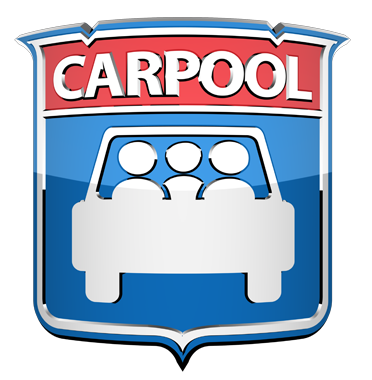# NCP Carpool Connection!

If you're interested in carpooling to school, add your address to the 2019-2020 NCP Carpool map and reach out to contact a potential carpool family on the map. Find the map HERE or on the Welcome page of FON Connect.

 table div table+table+table+table+table+table+table+table+table+table+table+table+table+table+table+table+table+table+table+table+table+table+table+table+table+table+table+table+table+table+table+table+table+table+table+table+table+table+table+table+table+table+table+table+table+table+table+table+table+table+table+table+table+table+table+table+table+table+table+table+table+table+table+table+table+table+table+table div table{width:100%;padding:0}table div table+table+table+table+table+table+table+table+table+table+table+table+table+table+table+table+table+table+table+table+table+table+table+table+table+table+table+table+table+table+table+table+table+table+table+table+table+table+table+table+table+table+table+table+table+table+table+table+table+table+table+table+table+table+table+table+table+table+table+table+table+table+table+table+table+table+table+table div table img{width:96.23%;padding:0;float:none}table div table+table+table+table+table+table+table+table+table+table+table+table+table+table+table+table+table+table+table+table+table+table+table+table+table+table+table+table+table+table+table+table+table+table+table+table+table+table+table+table+table+table+table+table+table+table+table+table+table+table+table+table+table+table+table+table+table+table+table+table+table+table+table+table+table+table+table+table div table td{width:100%;padding:0 1.88% 18px}/* styles */# Attention, Parents of CTA Commuters

Did you know that the #82 and #92 buses pick up students in front of Northside at the end of the school day? The Kimball Brown line station is also close to school at Kimball and Lawrence.

These are just a few of the ways students can use public transportation to commute to school. More information on routes for your student can be found at the CTA website HERE.

 table div table+table+table+table+table+table+table+table+table+table+table+table+table+table+table+table+table+table+table+table+table+table+table+table+table+table+table+table+table+table+table+table+table+table+table+table+table+table+table+table+table+table+table+table+table+table+table+table+table+table+table+table+table+table+table+table+table+table+table+table+table+table+table+table+table+table+table+table+table+table div table{width:100%;padding:0}table div table+table+table+table+table+table+table+table+table+table+table+table+table+table+table+table+table+table+table+table+table+table+table+table+table+table+table+table+table+table+table+table+table+table+table+table+table+table+table+table+table+table+table+table+table+table+table+table+table+table+table+table+table+table+table+table+table+table+table+table+table+table+table+table+table+table+table+table+table+table div table img{width:96.23%;padding:0;float:none}table div table+table+table+table+table+table+table+table+table+table+table+table+table+table+table+table+table+table+table+table+table+table+table+table+table+table+table+table+table+table+table+table+table+table+table+table+table+table+table+table+table+table+table+table+table+table+table+table+table+table+table+table+table+table+table+table+table+table+table+table+table+table+table+table+table+table+table+table+table+table div table td{width:100%;padding:0 1.88% 18px}/* styles */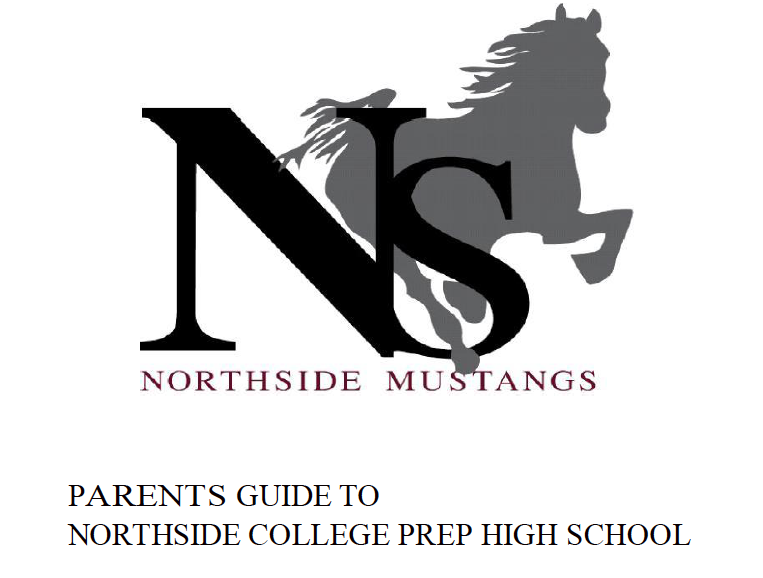# Navigating Northside

Navigating Northside, our parent handbook, is available on the school website under the "Parents" tab. Click HERE to access it directly.

 table div table+table+table+table+table+table+table+table+table+table+table+table+table+table+table+table+table+table+table+table+table+table+table+table+table+table+table+table+table+table+table+table+table+table+table+table+table+table+table+table+table+table+table+table+table+table+table+table+table+table+table+table+table+table+table+table+table+table+table+table+table+table+table+table+table+table+table+table+table+table+table+table div table{width:100%;padding:0}table div table+table+table+table+table+table+table+table+table+table+table+table+table+table+table+table+table+table+table+table+table+table+table+table+table+table+table+table+table+table+table+table+table+table+table+table+table+table+table+table+table+table+table+table+table+table+table+table+table+table+table+table+table+table+table+table+table+table+table+table+table+table+table+table+table+table+table+table+table+table+table+table div table img{width:96.23%;padding:0;float:none}table div table+table+table+table+table+table+table+table+table+table+table+table+table+table+table+table+table+table+table+table+table+table+table+table+table+table+table+table+table+table+table+table+table+table+table+table+table+table+table+table+table+table+table+table+table+table+table+table+table+table+table+table+table+table+table+table+table+table+table+table+table+table+table+table+table+table+table+table+table+table+table+table div table td{width:100%;padding:0 1.88% 18px}/* styles */# Give Back to Northside

Purchase school and office supplies at Office Depot and OfficeMax and Northside can receive 5% back in credits or FREE supplies. Simply provide our school ID 70031171 at checkout, online, or in stores. There’s no cost to you!

 table div table+table+table+table+table+table+table+table+table+table+table+table+table+table+table+table+table+table+table+table+table+table+table+table+table+table+table+table+table+table+table+table+table+table+table+table+table+table+table+table+table+table+table+table+table+table+table+table+table+table+table+table+table+table+table+table+table+table+table+table+table+table+table+table+table+table+table+table+table+table+table+table+table+table div table{width:100%;padding:0}table div table+table+table+table+table+table+table+table+table+table+table+table+table+table+table+table+table+table+table+table+table+table+table+table+table+table+table+table+table+table+table+table+table+table+table+table+table+table+table+table+table+table+table+table+table+table+table+table+table+table+table+table+table+table+table+table+table+table+table+table+table+table+table+table+table+table+table+table+table+table+table+table+table+table div table img{width:96.23%;padding:0;float:none}table div table+table+table+table+table+table+table+table+table+table+table+table+table+table+table+table+table+table+table+table+table+table+table+table+table+table+table+table+table+table+table+table+table+table+table+table+table+table+table+table+table+table+table+table+table+table+table+table+table+table+table+table+table+table+table+table+table+table+table+table+table+table+table+table+table+table+table+table+table+table+table+table+table+table div table td{width:100%;padding:0 1.88% 18px}/* styles */# Support Northside - Shop AmazonSmile

AmazonSmile is a simple and automatic way to support Northside every time you shop.

Please note that our AmazonSmile name is Friends of "North" "Side" (North Side - two words at AmazonSmile).

 table div table+table+table+table+table+table+table+table+table+table+table+table+table+table+table+table+table+table+table+table+table+table+table+table+table+table+table+table+table+table+table+table+table+table+table+table+table+table+table+table+table+table+table+table+table+table+table+table+table+table+table+table+table+table+table+table+table+table+table+table+table+table+table+table+table+table+table+table+table+table+table+table+table+table+table+table div table{width:100%;padding:0}table div table+table+table+table+table+table+table+table+table+table+table+table+table+table+table+table+table+table+table+table+table+table+table+table+table+table+table+table+table+table+table+table+table+table+table+table+table+table+table+table+table+table+table+table+table+table+table+table+table+table+table+table+table+table+table+table+table+table+table+table+table+table+table+table+table+table+table+table+table+table+table+table+table+table+table+table div table img{width:96.23%;padding:0;float:none}table div table+table+table+table+table+table+table+table+table+table+table+table+table+table+table+table+table+table+table+table+table+table+table+table+table+table+table+table+table+table+table+table+table+table+table+table+table+table+table+table+table+table+table+table+table+table+table+table+table+table+table+table+table+table+table+table+table+table+table+table+table+table+table+table+table+table+table+table+table+table+table+table+table+table+table+table div table td{width:100%;padding:0 1.88% 18px}/* styles */# Follow Us on Social Media

▪ Northside Prep Parents Facebook Page, a closed group for parents, by parents, to share their knowledge of Northside
▪ Friends of Northside Facebook Page, for news everyone can view
 ▪ Northside Prep Parents Facebook Page, a closed group for parents, by parents, to share their knowledge of Northside
 ▪ Friends of Northside Facebook Page, for news everyone can view
 table div table+table+table+table+table+table+table+table+table+table+table+table+table+table+table+table+table+table+table+table+table+table+table+table+table+table+table+table+table+table+table+table+table+table+table+table+table+table+table+table+table+table+table+table+table+table+table+table+table+table+table+table+table+table+table+table+table+table+table+table+table+table+table+table+table+table+table+table+table+table+table+table+table+table+table+table+table+table div table{width:100%;padding:0}table div table+table+table+table+table+table+table+table+table+table+table+table+table+table+table+table+table+table+table+table+table+table+table+table+table+table+table+table+table+table+table+table+table+table+table+table+table+table+table+table+table+table+table+table+table+table+table+table+table+table+table+table+table+table+table+table+table+table+table+table+table+table+table+table+table+table+table+table+table+table+table+table+table+table+table+table+table+table div table img{width:96.23%;padding:0;float:none}table div table+table+table+table+table+table+table+table+table+table+table+table+table+table+table+table+table+table+table+table+table+table+table+table+table+table+table+table+table+table+table+table+table+table+table+table+table+table+table+table+table+table+table+table+table+table+table+table+table+table+table+table+table+table+table+table+table+table+table+table+table+table+table+table+table+table+table+table+table+table+table+table+table+table+table+table+table+table div table td{width:100%;padding:0 1.88% 18px}/* styles */Northside’s website can be found HERE.

Friends of Northside's website can be found HERE.

 table div table+table+table+table+table+table+table+table+table+table+table+table+table+table+table+table+table+table+table+table+table+table+table+table+table+table+table+table+table+table+table+table+table+table+table+table+table+table+table+table+table+table+table+table+table+table+table+table+table+table+table+table+table+table+table+table+table+table+table+table+table+table+table+table+table+table+table+table+table+table+table+table+table+table+table+table+table+table+table+table div table{width:100%;padding:0}table div table+table+table+table+table+table+table+table+table+table+table+table+table+table+table+table+table+table+table+table+table+table+table+table+table+table+table+table+table+table+table+table+table+table+table+table+table+table+table+table+table+table+table+table+table+table+table+table+table+table+table+table+table+table+table+table+table+table+table+table+table+table+table+table+table+table+table+table+table+table+table+table+table+table+table+table+table+table+table+table div table img{width:96.23%;padding:0;float:none}table div table+table+table+table+table+table+table+table+table+table+table+table+table+table+table+table+table+table+table+table+table+table+table+table+table+table+table+table+table+table+table+table+table+table+table+table+table+table+table+table+table+table+table+table+table+table+table+table+table+table+table+table+table+table+table+table+table+table+table+table+table+table+table+table+table+table+table+table+table+table+table+table+table+table+table+table+table+table+table+table div table td{width:100%;padding:0 1.88% 18px}/* styles */